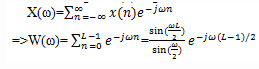Test: DFT Signal Analysis

# Test: DFT Signal Analysis

Test Description

## 10 Questions MCQ Test Signals and Systems | Test: DFT Signal Analysis

Test: DFT Signal Analysis for Electrical Engineering (EE) 2023 is part of Signals and Systems preparation. The Test: DFT Signal Analysis questions and answers have been prepared according to the Electrical Engineering (EE) exam syllabus.The Test: DFT Signal Analysis MCQs are made for Electrical Engineering (EE) 2023 Exam. Find important definitions, questions, notes, meanings, examples, exercises, MCQs and online tests for Test: DFT Signal Analysis below.
Solutions of Test: DFT Signal Analysis questions in English are available as part of our Signals and Systems for Electrical Engineering (EE) & Test: DFT Signal Analysis solutions in Hindi for Signals and Systems course. Download more important topics, notes, lectures and mock test series for Electrical Engineering (EE) Exam by signing up for free. Attempt Test: DFT Signal Analysis | 10 questions in 10 minutes | Mock test for Electrical Engineering (EE) preparation | Free important questions MCQ to study Signals and Systems for Electrical Engineering (EE) Exam | Download free PDF with solutions
 1 Crore+ students have signed up on EduRev. Have you?
Test: DFT Signal Analysis - Question 1

### If the signal to be analyzed is an analog signal, we would pass it through an anti-aliasing filter with B as the bandwidth of the filtered signal and then the signal is sampled at a rate:

Detailed Solution for Test: DFT Signal Analysis - Question 1

Explanation: The filtered signal is sampled at a rate of Fs≥ 2B, where B is the bandwidth of the filtered signal to prevent aliasing.

Test: DFT Signal Analysis - Question 2

### What is the highest frequency that is contained in the sampled signal?

Detailed Solution for Test: DFT Signal Analysis - Question 2

Explanation: We know that, after passing the signal through anti-aliasing filter, the filtered signal is sampled at a rate of Fs≥ 2B=>B≤ Fs/2.Thus the maximum frequency of the sampled signal is Fs/2.

Test: DFT Signal Analysis - Question 3

### The finite observation interval for the signal places a limit on the frequency resolution.

Detailed Solution for Test: DFT Signal Analysis - Question 3

Explanation: After sampling the signal, we limit the duration of the signal to the time interval T0=LT, where L is the number of samples and T is the sample interval. So, it limits our ability to distinguish two frequency components that are separated by less than 1/T0=1/LT in frequency. So, the finite observation interval for the signal places a limit on the frequency resolution.

Test: DFT Signal Analysis - Question 4

If {x(n)} is the signal to be analyzed, limiting the duration of the sequence to L samples, in the interval 0≤ n≤ L-1, is equivalent to multiplying {x(n)} by:

Detailed Solution for Test: DFT Signal Analysis - Question 4

Explanation: The equation of the rectangular window w(n) is given as
w(n)= 1, 0≤ n≤ L-1
=0, otherwise
Thus, we can limit the duration of the signal x(n) to L samples by multiplying it with a rectangular window of length L.

Test: DFT Signal Analysis - Question 5

What is the Fourier transform of rectangular window of length L?

Detailed Solution for Test: DFT Signal Analysis - Question 5

Explanation: We know that the equation for the rectangular window w(n) is given as
w(n)= 1, 0≤ n≤ L-1
=0, otherwise
We know that the Fourier transform of a signal x(n) is given asTest: DFT Signal Analysis - Question 6

If x(n)=cosω0n and W(ω) is the Fourier transform of the rectangular signal w(n), then what is the Fourier transform of the signal x(n).w(n)?

Detailed Solution for Test: DFT Signal Analysis - Question 6

Explanation: According to the exponential properties of Fourier transform, we get
Fourier transform of x(n).w(n)= 1/2[W(ω-ω0)+ W(ω+ω0)]

Test: DFT Signal Analysis - Question 7

The characteristic of windowing the signal called “Leakage” is the power that is leaked out into the entire frequency range.

Detailed Solution for Test: DFT Signal Analysis - Question 7

Explanation: We note that the windowed spectrum X ̂(ω) is not localized to a single frequency, but instead it is spread out over the whole frequency range. Thus the power of the original signal sequence x(n) that was concentrated at a single frequency has been spread by the window into the entire frequency range. We say that the power has been leaked out into the entire frequency range and this phenomenon is called as “Leakage”.

Test: DFT Signal Analysis - Question 8

Which of the following is the advantage of Hanning window over rectangular window?

Detailed Solution for Test: DFT Signal Analysis - Question 8

Explanation: The Hanning window has less side lobes and the leakage is less in this windowing technique.

Test: DFT Signal Analysis - Question 9

Which of the following is the disadvantage of Hanning window over rectangular window?

Detailed Solution for Test: DFT Signal Analysis - Question 9

Explanation: In the magnitude response of the signal windowed using Hanning window, the width of the main lobe is more which is the disadvantage of this technique over rectangular windowing technique.

Test: DFT Signal Analysis - Question 10

The condition with less number of samples L should be avoided.

Detailed Solution for Test: DFT Signal Analysis - Question 10

Explanation: When the number of samples L is small, the window spectrum masks the signal spectrum and, consequently , the DFT of the data reflects the spectral characteristics of the window function. So, this situation should be avoided.

## Signals and Systems

32 videos|76 docs|63 tests
 Use Code STAYHOME200 and get INR 200 additional OFF Use Coupon Code
Information about Test: DFT Signal Analysis Page
In this test you can find the Exam questions for Test: DFT Signal Analysis solved & explained in the simplest way possible. Besides giving Questions and answers for Test: DFT Signal Analysis, EduRev gives you an ample number of Online tests for practice

## Signals and Systems

32 videos|76 docs|63 tests